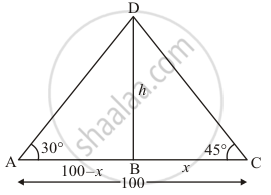# Two Boats Approach a Light House in Mid-sea from Opposite Directions. the Angles of Elevation of the Top of the Light House from Two Boats Are 30° and 45° Respectively. If the Distance Between Two Boats is 100 M, Find the Height of the Light House. - Mathematics

Two boats approach a lighthouse in mid-sea from opposite directions. The angles of elevation of the top of the lighthouse from two boats are 30° and 45° respectively. If the distance between two boats is 100 m, find the height of the lighthouse.

#### Solution

Let h be the height of lighthouse BD. An angle of elevation of the top of the lighthouse from two boats is 30° and 45°.

Let DB = h , BC = x and it is given that AC = 100 m. So

AB = 100 - x. And ∠DAB = 30°, ∠BCD = 45°

Here we have to find height of light house.

The corresponding figure is as followsSo we use trigonometric ratios.

In ΔBDC

=> tan  45° = (BD)/(BC)

=> 1 = h/x

=> x = h

Again in  ΔDAB

=> tan 30° = (DB)/(AB)

=> 1/sqrt3 = h/(100 - x)

=> sqrt3h = 100 - x

=> (sqrt3 + 1)h = 100

=> h = 100/(sqrt3 + 1)  xx (sqrt3 - 1)/(sqrt3 - 1)

=> h = 50(sqrt3 - 1)

Hence the height of light house is 50(sqrt3 - 1) m

Concept: Heights and Distances
Is there an error in this question or solution?
Chapter 12: Trigonometry - Exercise 12.1 [Page 33]

#### APPEARS IN

RD Sharma Class 10 Maths
Chapter 12 Trigonometry
Exercise 12.1 | Q 60 | Page 33

Share